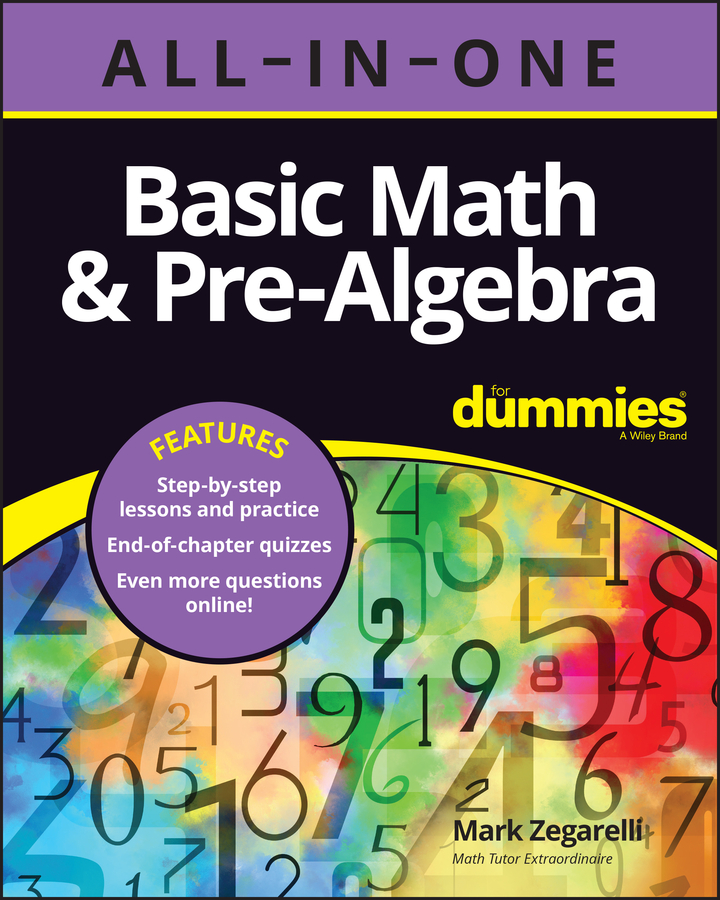##### Basic Math & Pre-Algebra All-in-One For Dummies (+ Chapter Quizzes Online)Word problems help you understand the logic behind setting up equations in real-life situations. The skill you need most when working with word problems is understanding how to turn the situation you’re facing into numbers you can calculate.

Generally speaking, converting a word problem into an equation involves reading through the problem and setting up word equations — that is, equations that contain words as well as numbers.

Most word problems give you information about numbers, telling you exactly how much, how many, how fast, how big, and so forth. Here are some examples:

Nunu is spinning 17 plates.

The width of the house is 80 feet.

If the local train is going 25 miles per hour . . .

You need this information to solve the problem. And paper is cheap, so don’t be afraid to use it. (If you’re concerned about trees, write on the back of all that junk mail you get.) Have a piece of scrap paper handy and jot down a few notes as you read through a word problem.

For example, here’s how you can jot down “Nunu is spinning 17 plates”:

Nunu = 17

Here’s how to note that “. . . the width of the house is 80 feet”:

Width = 80

The third example tells you, “If the local train is going 25 miles per hour. . . .” So you can jot down the following:

Local = 25

Don’t let the word if confuse you. When a problem says “If so-and-so were true . . .” and then asks you a question, assume it is true and use this information to answer the question.

When you jot down information this way, you’re really turning words into a more useful form called a word equation. A word equation has an equal sign like a math equation, but it contains both words and numbers.

When you start doing word problems, you notice that certain words and phrases show up over and over again. For example,

Bobo is spinning five fewer plates than Nunu.

The height of a house is half as long as its width.

The express train is moving three times faster than the local train.

You’ve probably seen statements such as these in word problems since you were first doing math. Statements like these look like English, but they’re really math, so spotting them is important. You can represent each of these types of statements as word equations that also use Big Four operations (addition, subtraction, division, and multiplication). Look again at the first example:

Bobo is spinning five fewer plates than Nunu.

You don’t know the number of plates that either Bobo or Nunu is spinning. But you know that these two numbers are related.

You can express this relationship like this:

Bobo = Nunu – 5

This word equation is shorter than the statement it came from.

Here’s another example:

The height of a house is half as long as its width.

You don’t know the width or height of the house, but you know that these numbers are connected.

You can express this relationship between the width and height of the house as the following word equation:

Height = width ÷ 2

With the same type of thinking, you can translate The express train is moving three times faster than the local train into this word equation:

Express = 3 · local

As you can see, each of the examples allows you to set up a word equation using one of the Big Four operations.

The end of a word problem usually contains the question that you need to answer to solve the problem. You can use word equations to clarify this question so you know right from the start what you’re looking for.

For example, you can write the question, “All together, how many plates are Bobo and Nunu spinning?” as

Bobo + Nunu = ?

You can write the question “How tall is the house” as:

Height = ?

Finally, you can rephrase the question “What’s the difference in speed between the express train and the local train?” in this way:

Express – Local = ?

At this point, you can plug in numbers to solve the word problem.